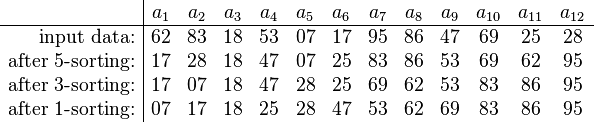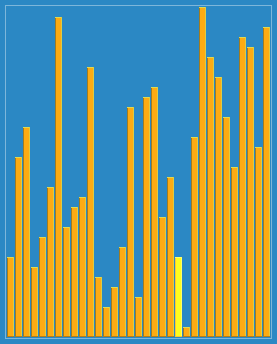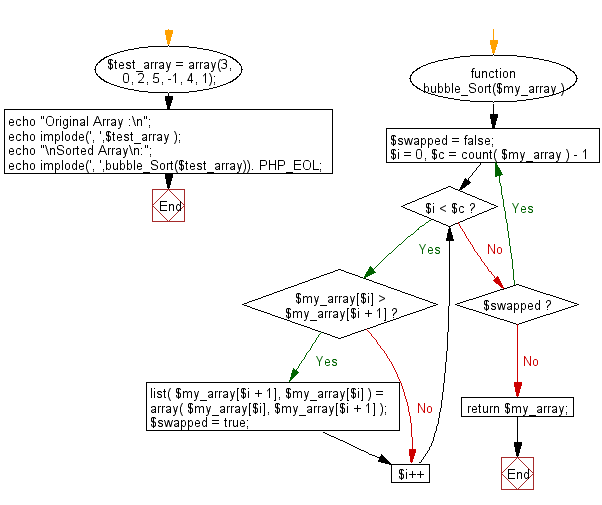# JavaScript Sorting Algorithm: Shell sort

## JavaScript Sorting Algorithm: Exercise-6 with Solution

Write a JavaScript program to sort a list of elements using Shell sort.

According to Wikipedia "Shell sort or Shell's method, is an in-place comparison sort. It can be seen as either a generalization of sorting by exchange (bubble sort) or sorting by insertion (insertion sort). The method starts by sorting pairs of elements far apart from each other, then progressively reducing the gap between elements to be compared. Starting with far apart elements can move some out-of-place elements into position faster than a simple nearest neighbor exchange."

An example run of Shellsort with gaps 5, 3 and 1 is shown below.The first pass, 5-sorting, performs insertion sort on separate subarrays (a1, a6, a11), (a2, a7, a12), (a3, a8), (a4, a9), (a5, a10). For instance, it changes the subarray (a1, a6, a11) from (62, 17, 25) to (17, 25, 62). The next pass, 3-sorting, performs insertion sort on the subarrays (a1, a4, a7, a10), (a2, a5, a8, a11), (a3, a6, a9, a12). The last pass, 1-sorting, is an ordinary insertion sort of the entire array (a1,..., a12). As the example illustrates, the subarrays that Shellsort operates on are initially short; later they are longer but almost ordered. In both cases insertion sort works efficiently. Shellsort is unstable: it may change the relative order of elements with equal values. It is an adaptive sorting algorithm in that it executes faster when the input is partially sorted.

Shell SortShellsort with gaps 23, 10, 4, 1 in action.

Sample Solution:

JavaScript Code:

``````function shellSort(arr) {
var increment = arr.length / 2;
while (increment > 0) {
for (i = increment; i < arr.length; i++) {
var j = i;
var temp = arr[i];

while (j >= increment && arr[j-increment] > temp) {
arr[j] = arr[j-increment];
j = j - increment;
}

arr[j] = temp;
}

if (increment == 2) {
increment = 1;
} else {
increment = parseInt(increment*5 / 11);
}
}
return arr;
}

console.log(shellSort([3, 0, 2, 5, -1, 4, 1]));
```
```

Sample Output:

```[-1,0,1,2,3,4,5]
```

Flowchart:Live Demo:

See the Pen searching-and-sorting-algorithm-exercise-6 by w3resource (@w3resource) on CodePen.

Improve this sample solution and post your code through Disqus

What is the difficulty level of this exercise?

Test your Programming skills with w3resource's quiz.

﻿